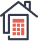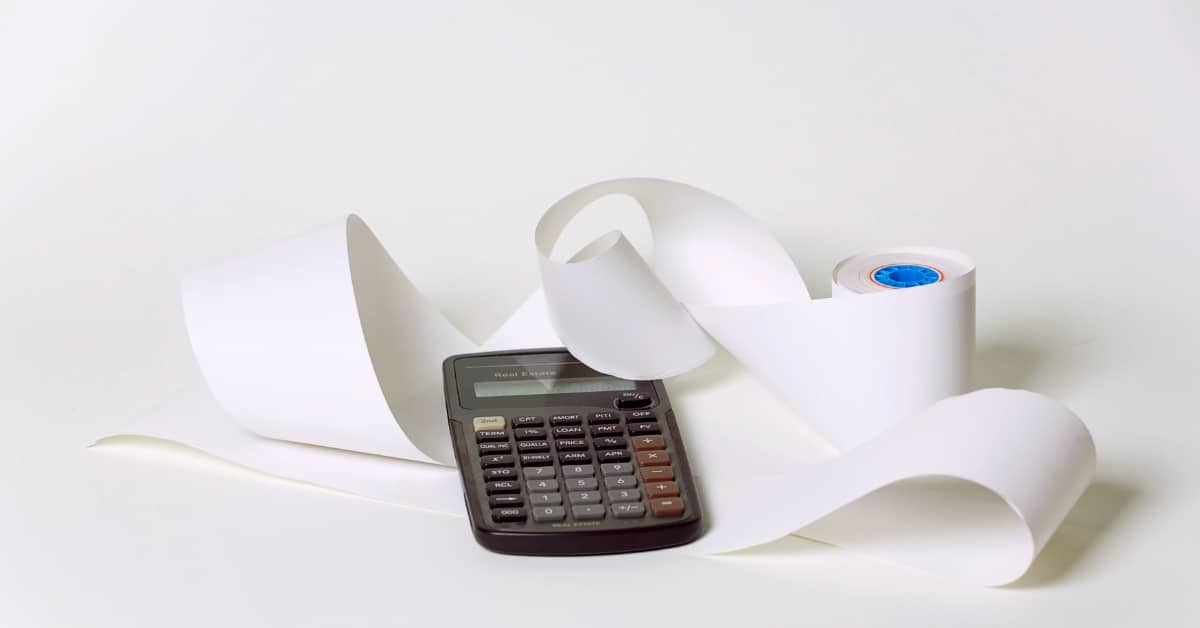Rental
Property Calculator

# DSCR Loans Formula: What is DSCR and How Does It Work?By:
Amy Gilmore
Updated
November 18, 2022The DSCR loan formula is the debt service credit ratio. It is a figure that real estate investment businesses, lenders, and government entities use to calculate the cash flow available to pay loan principal and interest (debt service) for a year or other period.

Read this guide to learn how to calculate DSCR, what you use it for, and how it works. It includes formulas and valuable information to help you use DSCR to benefit your growing real estate investment business.

## What is Debt Service Coverage Ratio Loan Formula (DSCR)?

The formula for DSCR is net operating income (NOI) divided by the debt service (DS) of a loan. To calculate the DSCR for the year, you would use the total net operating income divided by the total debt service for the year.

For example:

• If the total net operating income is \$20,000 and the debt service is \$14,400, the DSCR is 1.38.
• When the total NOI is \$20,000 and the debt service is \$10,000, the DSCR is 2.

## Who Uses DSCR?

Real estate investment firms, lenders, and government agencies use DSCR to calculate an applicant or borrower’s ability to manage loan debt. The lower the DSCR, the less cash flow is available to pay the debt service.In addition to DSCR, lenders use debt-to-income ratio, credit scores, loan-to-value ratio, and other figures to determine whether you qualify for a loan.

## Why Should You Learn the DSCR Loans Formula?

DSCR is a figure that lenders use, so if you want to see where you stand financially in the eyes of lenders, these calculations are beneficial. You should also consider other figures when determining whether a property is a good investment.

You should also calculate the cap rate, internal rate of return (IRR), closing cost, cash to close, and total return on investment (ROI) if you are trying to determine the value of an investment property.

## How Do You Calculate DSCR?

Determining DSCR requires you to calculate a few figures. Here are the steps you need to follow.### 1. Determine the Period

You need to determine the period for which you will calculate DSCR. You could do this for a month or the entire year. However, it is most common for people to calculate DSCR for the entire year.

### 2. Calculate the Net Operating Income (NOI)

Net operating income is the total income generated by a property after factoring in any expenses. If you are calculating the NOI for the year, add all of the income that the property generates during that period and subtract the cost of managing and maintaining the property.

Some of the costs you need to subtract from the gross operating income include:

• HOA Payments
• Property Tax Obligations
• Routine Maintenance Costs
• Any Other Management Costs

### 3. Calculate the Debt Service

Calculating debt service is a bit more complicated because lenders and entities use different formulas. If you want to use the same debt service calculation as your lender, you can ask your loan officer for that information.

Otherwise, you can calculate detailed DS by using the formula below:

• (Interest x (1 - Tax Rate)) + Principal)

This is the most accurate way to calculate DS because interest is tax deductible while the principal is not.

### 4. Divide the NOI

Once you determine the DS and NOI, you divide the NOI by the DS to determine the total DSCR.

## Example DSCR Formulas

Here are a few examples of the DSCR formula. However, you can also use the DSCR tool on rentalpropertycalculator.com.### Monthly DSCR

Here are a few examples of monthly DSCR calculations:

• If the monthly NOI is \$1,500 per month and the DS is \$1,350, the formula is \$1,500 ➗\$1,350 = 1.11.
• With a monthly NOI of \$2,000 per month and a DS of \$1,500, the formula is \$2,000 ➗\$1,500 = 1.33.

### Annual DSCR

If you are calculating for the entire year, you multiply the monthly income and DS by 12 to determine the figures for the calculation. Here are a couple of examples:

• When the yearly NOI is \$15,000, and the DS is \$13,000, the formula is \$15,000 ➗\$13,000 = 1.15.
• If the NOI is \$24,000 and the DS is \$17,500, the formula is \$24,000 ➗\$17,500 = 1.37

## How Does DSCR Work?

The DSCR formula gives you a number that you can use to determine the cash flow you have available to pay your loan. You could also use DSCR to compare properties you may want to buy.A favorable DSCR is above 1.5, and lenders and investors typically require a DSCR of 1.22 or higher.

## Final Advice on DSCR Loans Formula

The DSCR loans formula is a simple way of determining someone’s ability to cover their loan debt expenses. While you can follow the formula in this guide to calculate DSCR, you can also determine DSCR by using the calculator on rentalpropertycalculator.com.Written By:
Amy Gilmore
Amy has over ten years of experience as a title company branch manager and closing trainer. Her escrow book of business includes national lenders, real estate wholesalers, investors, property developers, and top-selling realtors. Amy is passionate about teaching the benefits of investing in property and mentoring others in the real estate industry.